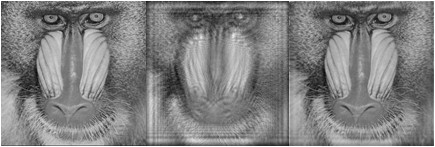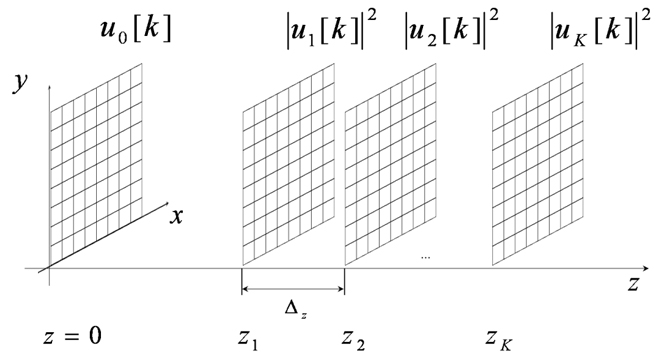# Inverse wave field imagingMulti-plane phase retrievalForward/backward modelingMulti-plane phase retrievalSparse reconstruction Publications Contacts

In our wave field propagation model the result of the forward diffraction propagetion is detected with a sensor at different planes, at various distances from the object and parallel sensor (image) plane z_r. The conventional sensor can detect only the intensity of the light, and the phase is systematically lost in the mesuarements. The phase carries information about the object shape, and recovering of the phase is of important to reconstruct the object amplitude and phase. Here we present a multi-plane parallel iterative phase-retrieval algorithm from a number of (noisy) intensity observation based on the augmented Lagrangian (AL) technique. All modeling can be done with the presented demo m-files demoAL#.m, realising different optical scenarios of the propagation. These scripts return the estimate of the object wave field distribution by the AL phase-retrieval methods and (for comparison) by the successive phase-retrival algorithm "single-beam multiple-intensity phase reconstruction" (SBMIR). The accuracy of the reconstructed object amplitude and phase is represented via the root-mean-square error (RMSE). This demo works with gray-scale or binary images only (a color image will be converted to a gray-scale one automatically).

The LASIP routines are available free-of-charge for educational and non-profit scientific research, enabling others researchers to understand and reproduce our work. Any unauthorized use of the LASIP routines for industrial or profit-oriented activities is expressively prohibited. Please read the LASIP limited licensebefore you proceed with downloading the files.

 CLICK HERE TO DOWNLOAD .ZIP PACKAGEAugmented Lagrangian (AL)Fig. 2.1. Multiple plane wave field reconstruction scenario: and are discrete complex amplitudes at the object and measurement planes respectively,

The main idea is that the object is illuminated with a plane laser beam, it scatteres from the object, and the result of the wave field propagation is detected on a sensor at different distances. So the volume of the wave fields is generated by the 2D object distribution . The non-interferometric (phase-retrieval) methods allow reconstructing the amplitude and phase of an object wave field iteratively on a computer from a number of noisy intensity observations. Thus we can represent the three dimensional object: the magnitude of the object distribution and recalculate the phase into the depth. The phase retrieval approach is much simpler than the conventional interferometric ones (there is no reference beam), and phase-retrieval techniques are much more robust to different disturbances.
In this work we assume that the wave field distributions at the object and measurement planes are pixilated. We consider a discrete-to-discrete model as in Section Forward/backward modeling. The link between the planes is defined in the vector-matrix notation as follows:

where is an arbitrary wave field propagation operator (for instance, F-DDT or the conventional angular spectrum decomposition ASD). In our model we have only noisy intensity observations from the sensor planes, which are given as follos:

where is an additive Gaussian noise. It means we have to recover the phase at the sensor planes and reconstruct the object wave field distribution using a number of observations. We assume that , where is a constant distance between the measurement planes.
We do not follow any variant of Gerchberg-Saxton or Fienup's error-reduction algorithms but rather apply the maximum likelihood style approach. The wave field reconstruction is formulated as the following optimization problem:

subject to (the forward propagation is considered as a constraint). Here μ is a regularization parameter, which controls a balance between the accuracy of the observation fitting and prior information on the object. The augmented Lagrangian (AL) technique is used for the minimization of the functionals in presence of linear equality constraints. Then the object wave field is reconstructed by optimization of the following criterion (Eq. 15 in ):

Here ()H denotes the complex conjugate transpose. The Lagrangian based optimization is associated with the saddle problem, which requires minimization on , and maximization on the vector of the Lagrange multipliers . The parameters are positive. The proposed algorithm corresponds with an iterative optimization of the criterion separately on , and (Eqs. 18-20 in ). For the object distribution of an arbitrary form its estimate can be found from the minimum condition, and we arrive at the proposed AL phase-retrival algorithm:

0. Initialization for t=0 : ,
Repear for t=1,2,...

End on t
This algorithm calculates the estimate of the object wave field distribution as
In Step 1 we calculate an estimate for the sensor plane wave field, and further update it in Step 2, where the non-linear algorithm (see Eq. A5 in ) returns a solution of minimization of L on as the best fit to the given observation (what is different with the conventional change of the magnitude/phase). Step 3 updates the Lagrangian multipliers as the difference between the initial and updated estimates of . In Step 4 we calculate the reconstruction of the object wave field distribution, used in Step 1 for prediction of the wave fields at sensor planes.

## References and publicationsA. Migukin, V. Katkovnik, and J. Astola, “Wave field reconstruction from multiple plane intensity-only data: augmented Lagrangian algorithm,” J. Opt. Soc. Am. A 28, 993-1002 (2011).A.Migukin, V. Katkovnik, J. Astola, “Optimal phase retrieval from multiple observations with Gaussian noise: augmented Lagrangian algorithm for phase objects”, SPIE Conf. 2011.A.Migukin, V. Katkovnik, J. Astola, “Phase retrieval from multiple plane observations: constrained variational formalization and augmanted Lagrangian recursive algorithm”, 2010 Workshop on Information Theoretic Methods in Science and Engineering, WITMSE'2010, Tampere, Finland, 2010.
 V. Katkovnik, A.Migukin, J. Astola, “3D wave field reconstruction from intensity-only data: Variational inverse imaging techniques”, 9th Euro-American Workshop on Information Optics, WIO'2010, Helsinki, Finland, 2010.A. Migukin, V. Katkovnik, and J. Astola, “3D Wave field phase retrieval from multi-plane observations”, IEEE Conf. 3DTV-CON, 2010.A. Migukin, V. Katkovnik, and J. Astola, “Multiple plane phase retrieval based on inverse regularized imaging and discrete diffraction transform,” AIP Conf. Proc.1236, ICAPMMOI, 2010.